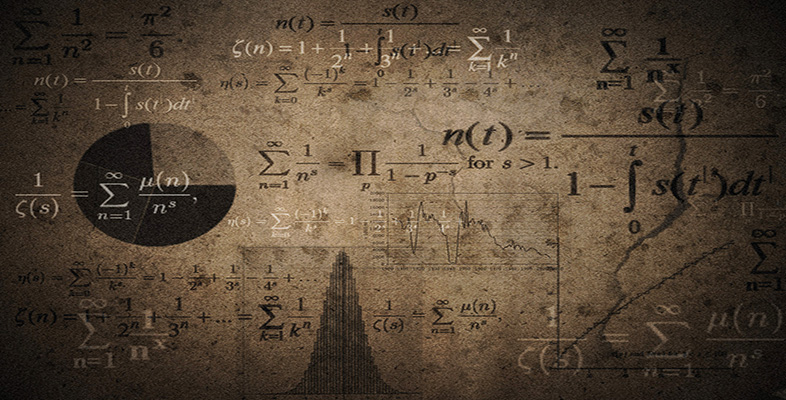Language, notation and formulas

This free course is available to start right now. Review the full course description and key learning outcomes and create an account and enrol if you want a free statement of participation.

Free course

# Language, notation and formulas

## 2.1 Layout

As mentioned in the animation in Section 1.2 writing mathematics has a lot in common with writing English. When you write mathematics, you should write in the equivalent of sentences, with full stops at the end. As in English, each new statement should follow on logically from the previous one or it should contain an indication that a new idea is being introduced. However, laying out mathematics differs from laying out English: because mathematics is more condensed than written English, it is often best to start each statement on a new line so that the various steps involved are readily apparent.

In writing mathematics you can use a mixture of words and symbols – symbols in mathematics are just shorthand for words. If you do not know the correct symbol, use the word itself.

Above all, make sure that you write so that somebody else can understand – that somebody might be yourself at a later date! It often helps to read aloud the mathematics that you have written to see if it makes sense.

### Example 1

• (a) 24.67 − 12.45 = 12.22

• (b) 3 × 4 = 4× 3 = 12.

• (a) Twenty-four point six seven minus twelve point four five equals twelve point two two.

• (b) Three times four equals four times three which is equal to twelve.

MU120_4M6